# Ridge Regression in R Programming

Ridge regression is a classification algorithm that works in part as it doesn’t require unbiased estimators. Ridge regression minimizes the residual sum of squares of predictors in a given model. Ridge regression includes a shrinks the estimate of the coefficients towards zero.

Ridge regression is a regularized regression algorithm that performs L2 regularization that adds an L2 penalty, which equals the square of the magnitude of coefficients. All coefficients are shrunk by the same factor i.e none are eliminated. L2 regularization will not result in sparse models. Ridge regression adds bias to make the estimates reliable approximations to true population values. Ridge regression proceeds by adding a small value k to the diagonal elements of the correlation matrix i.e ridge regression got its name since the diagonal of ones in the correlation matrix are thought to be a ridge.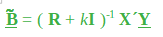Here, k is a positive quantity less than 1(usually less than 0.3). The amount of bias in estimator is given by: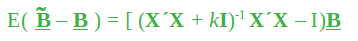The covariance matrix is given by: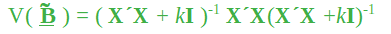There exists a value of k for which the Mean Squared Error(MSE i.e variance plus the bias squared) of the ridge estimator is less than least squares estimator. The appropriate value of k depends on the true regression coefficients(that are being estimated)and the optimality of the ridge solution.

• When lambda = 0, ridge regression equals least squares regression.
• When lambda = infinity, all coefficients shrunk to zero.

Also, the ideal penalty is in between 0 and infinity. Let’s implement Ridge regression in R programming.

### Implementation in R

##### The Dataset

Big Mart dataset consists of 1559 products across 10 stores in different cities. Certain attributes of each product and store have been defined. It consists of 12 features i.e Item_Identifier( is a unique product ID assigned to every distinct item), Item_Weight(includes the weight of the product), Item_Fat_Content(describes whether the product is low fat or not), Item_Visibility(mentions the percentage of the total display area of all products in a store allocated to the particular product), Item_Type(describes the food category to which the item belongs), Item_MRP(Maximum Retail Price (list price) of the product), Outlet_Identifier(unique store ID assigned. It consists of an alphanumeric string of length 6), Outlet_Establishment_Year(mentions the year in which store was established), Outlet_Size(tells the size of the store in terms of ground area covered), Outlet_Location_Type(tells about the size of the city in which the store is located), Outlet_Type(tells whether the outlet is just a grocery store or some sort of supermarket) and Item_Outlet_Sales( sales of the product in the particular store).

 `# Loading data ` `train = ``fread``(``"Train_UWu5bXk.csv"``) ` `test = ``fread``(``"Test_u94Q5KV.csv"``) ` `   `  `# Structure  ` `str``(train) `

Output: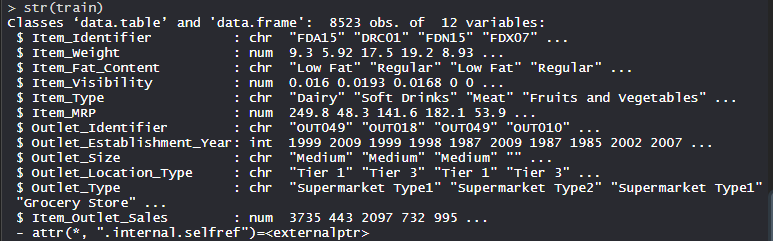##### Performing Ridge Regression on Dataset

Using Ridge regression algorithm on the dataset which includes 12 features with 1559 products across 10 stores in different cities.

 `# Installing Packages ` `install.packages``(``"data.table"``) ` `install.packages``(``"dplyr"``) ` `install.packages``(``"glmnet"``) ` `install.packages``(``"ggplot2"``) ` `install.packages``(``"caret"``) ` `install.packages``(``"xgboost"``) ` `install.packages``(``"e1071"``) ` `install.packages``(``"cowplot"``) ` ` `  `# load packages ` `library``(data.table) ``# used for reading and manipulation of data ` `library``(dplyr)      ``# used for data manipulation and joining ` `library``(glmnet)     ``# used for regression ` `library``(ggplot2)    ``# used for ploting  ` `library``(caret)      ``# used for modeling ` `library``(xgboost)    ``# used for building XGBoost model ` `library``(e1071)      ``# used for skewness ` `library``(cowplot)    ``# used for combining multiple plots  ` ` `  `# Loding datasets ` `train = ``fread``(``"Train_UWu5bXk.csv"``) ` `test = ``fread``(``"Test_u94Q5KV.csv"``) ` ` `  `# Setting test dataset ` `# Combining datasets ` `# add Item_Outlet_Sales to test data ` `test[, Item_Outlet_Sales := ``NA``] ` ` `  `combi = ``rbind``(train, test) ` ` `  `# Missing Value Treatment ` `missing_index = ``which``(``is.na``(combi\$Item_Weight)) ` `for``(i ``in` `missing_index) ` `{   ` `  ``item = combi\$Item_Identifier[i] ` `  ``combi\$Item_Weight[i] =  ` `         ``mean``(combi\$Item_Weight[combi\$Item_Identifier == item],  ` `         ``na.rm = T)   ` `} ` ` `  `# Replacing 0 in Item_Visibility with mean ` `zero_index = ``which``(combi\$Item_Visibility == 0) ` `for``(i ``in` `zero_index) ` `{   ` `  ``item = combi\$Item_Identifier[i] ` `  ``combi\$Item_Visibility[i] =  ` `        ``mean``(combi\$Item_Visibility[combi\$Item_Identifier == item], ` `                                                       ``na.rm = T)   ` `} ` ` `  `# Label Encoding ` `# To convert categorical in numerical ` `combi[, Outlet_Size_num := ``ifelse``(Outlet_Size == ``"Small"``, 0, ` `                             ``ifelse``(Outlet_Size == ``"Medium"``, 1, 2))] ` ` `  `combi[, Outlet_Location_Type_num :=  ` `                  ``ifelse``(Outlet_Location_Type == ``"Tier 3"``, 0, ` `                    ``ifelse``(Outlet_Location_Type == ``"Tier 2"``, 1, 2))] ` ` `  `combi[, ``c``(``"Outlet_Size"``, ``"Outlet_Location_Type"``) := ``NULL``] ` ` `  `# One Hot Encoding ` `# To convert categorical in numerical ` `ohe_1 = ``dummyVars``(``"~."``, data = combi[, -``c``(``"Item_Identifier"``,  ` `                                          ``"Outlet_Establishment_Year"``, ` `                                          ``"Item_Type"``)], fullRank = T) ` `ohe_df = ``data.table``(``predict``(ohe_1, combi[, -``c``(``"Item_Identifier"``,  ` `                                          ``"Outlet_Establishment_Year"``,  ` `                                          ``"Item_Type"``)])) ` ` `  `combi = ``cbind``(combi[, ``"Item_Identifier"``], ohe_df) ` ` `  `# Remove skewness ` `skewness``(combi\$Item_Visibility)  ` `skewness``(combi\$price_per_unit_wt) ` ` `  `# log + 1 to avoid division by zero ` `combi[, Item_Visibility := ``log``(Item_Visibility + 1)]  ` ` `  `# Scaling and Centering data ` `num_vars = ``which``(``sapply``(combi, is.numeric)) ``# index of numeric features ` `num_vars_names = ``names``(num_vars) ` ` `  `combi_numeric = combi[, ``setdiff``(num_vars_names, ``"Item_Outlet_Sales"``), ` `                                                           ``with = F] ` ` `  `prep_num = ``preProcess``(combi_numeric, method=``c``(``"center"``, ``"scale"``)) ` `combi_numeric_norm = ``predict``(prep_num, combi_numeric) ` ` `  `# removing numeric independent variables ` `combi[, ``setdiff``(num_vars_names, ``"Item_Outlet_Sales"``) := ``NULL``]  ` `combi = ``cbind``(combi, combi_numeric_norm) ` ` `  `# splitting data back to train and test ` `train = combi[1:``nrow``(train)] ` `test = combi[(``nrow``(train) + 1):``nrow``(combi)] ` ` `  `# Removing Item_Outlet_Sales ` `test[, Item_Outlet_Sales := ``NULL``]  ` ` `  `# Model Building :Lasso Regression ` `set.seed``(123) ` `control = ``trainControl``(method =``"cv"``, number = 5) ` `Grid_la_reg = ``expand.grid``(alpha = 1, lambda = ``seq``(0.001,  ` `                                       ``0.1, by = 0.0002)) ` ` `  `# Model Building : Ridge Regression ` `set.seed``(123) ` `control = ``trainControl``(method =``"cv"``, number = 5) ` `Grid_ri_reg = ``expand.grid``(alpha = 0, lambda = ``seq``(0.001, 0.1, ` `                                                ``by = 0.0002)) ` ` `  `# Training Ridge Regression model ` `Ridge_model = ``train``(x = train[, -``c``(``"Item_Identifier"``, ` `                                ``"Item_Outlet_Sales"``)], ` `                    ``y = train\$Item_Outlet_Sales, ` `                    ``method = ``"glmnet"``, ` `                    ``trControl = control, ` `                    ``tuneGrid = Grid_reg ` `                    ``) ` `Ridge_model ` ` `  `# mean validation score ` `mean``(Ridge_model\$resample\$RMSE) ` ` `  `# Plot ` `plot``(Ridge_model, main=``"Ridge Regression"``) `

Output:

• Model Ridge_model: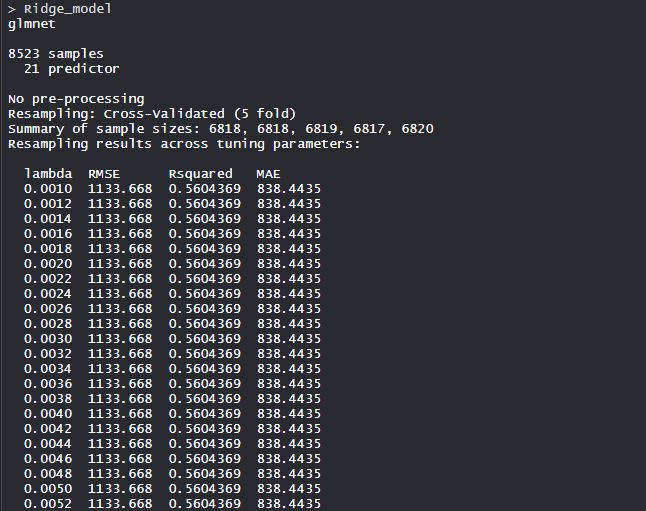The Ridge regression model uses the alpha value as 0 and lambda value as 0.1. RMSE was used to select the optimal model using the smallest value.

• Mean validation score: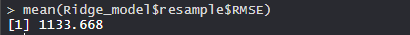The mean validation score of the model is 1133.668.

• Plot: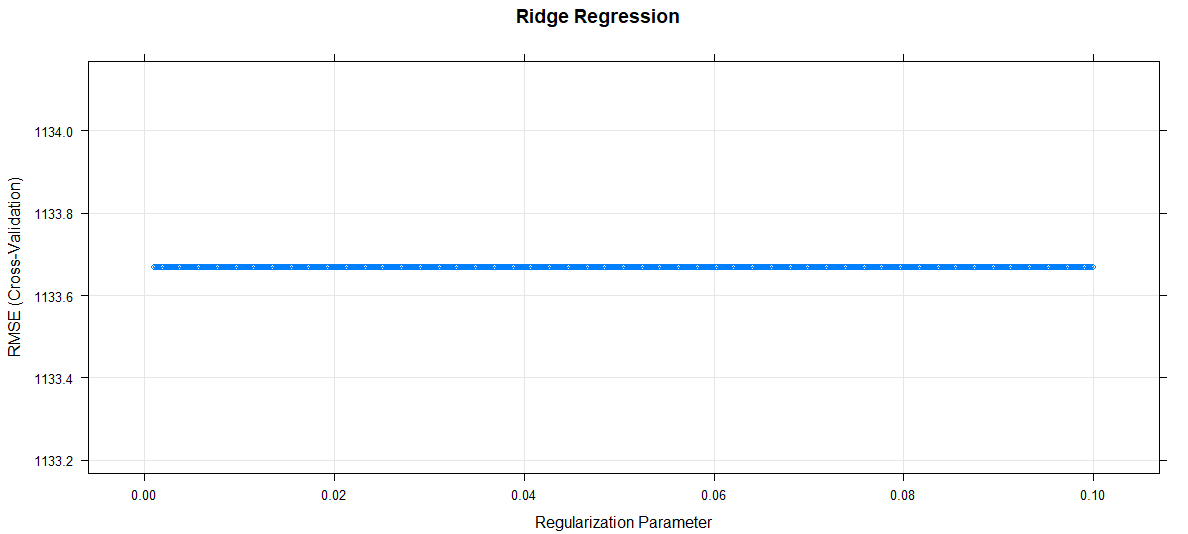The regularization parameter increases, RMSE remains constant.

• So, Ridge regression applications are used in many sectors of industry and with full capacity.

My Personal Notes arrow_drop_upCheck out this Author's contributed articles.

If you like GeeksforGeeks and would like to contribute, you can also write an article using contribute.geeksforgeeks.org or mail your article to contribute@geeksforgeeks.org. See your article appearing on the GeeksforGeeks main page and help other Geeks.

Please Improve this article if you find anything incorrect by clicking on the "Improve Article" button below.

Article Tags :

Be the First to upvote.

Please write to us at contribute@geeksforgeeks.org to report any issue with the above content.